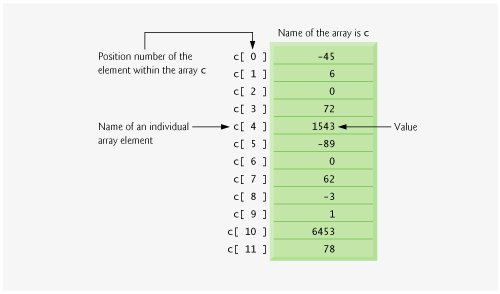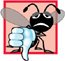# Arrays

An array is a consecutive group of memory locations that all have the same type. To refer to a particular location or element in the array, we specify the name of the array and the position number of the particular element in the array.

Figure 7.1 shows an integer array called c. This array contains 12 elements. A program refers to any one of these elements by giving the name of the array followed by the position number of the particular element in square brackets ([]). The position number is more formally called a subscript or index (this number specifies the number of elements from the beginning of the array). The first element in every array has subscript 0 (zero) and is sometimes called the zeroth element. Thus, the elements of array c are c[ 0 ] (pronounced "c sub zero"), c[ 1 ], c[ 2 ] and so on. The highest subscript in array c is 11, which is 1 less than 12the number of elements in the array. Array names follow the same conventions as other variable names, i.e., they must be identifiers.

Figure 7.1. Array of 12 elementsA subscript must be an integer or integer expression (using any integral type). If a program uses an expression as a subscript, then the program evaluates the expression to determine the subscript. For example, if we assume that variable a is equal to 5 and that variable b is equal to 6, then the statement

```c[ a + b ] += 2;
```

adds 2 to array element c[ 11 ]. Note that a subscripted array name is an lvalueit can be used on the left side of an assignment, just as non-array variable names can.

Let us examine array c in Fig. 7.1 more closely. The name of the entire array is c. The 12 elements of array c are referred to as c[ 0 ], c[ 1 ], c[ 2 ], ..., c[ 11 ]. The value of c[ 0 ] is -45, the value of c[ 1 ] is 6, the value of c[ 2 ] is 0, the value of c[ 7 ] is 62, and the value of c[ 11 ] is 78. To print the sum of the values contained in the first three elements of array c, we would write

```cout << c[ 0 ] + c[ 1 ] + c[ 2 ] << endl;
```

To divide the value of c[ 6 ] by 2 and assign the result to the variable x, we would write

```x = c[ 6 ] / 2;
```

Common Programming Error 7.1It is important to note the difference between the "seventh element of the array" and "array element 7." Array subscripts begin at 0, so the "seventh element of the array" has a subscript of 6, while "array element 7" has a subscript of 7 and is actually the eighth element of the array. Unfortunately, this distinction frequently is a source of off-by-one errors. To avoid such errors, we refer to specific array elements explicitly by their array name and subscript number (e.g., c[ 6 ] or c[ 7 ]).

The brackets used to enclose the subscript of an array are actually an operator in C++. Brackets have the same level of precedence as parentheses. Figure 7.2 shows the precedence and associativity of the operators introduced so far. Note that brackets ([]) have been added to the first row of Fig. 7.2. The operators are shown top to bottom in decreasing order of precedence with their associativity and type.

Figure 7.2. Operator precedence and associativity.

Operators

Associativity

Type

()

[]

left to right

highest

++

--

static_cast<type>( operand )

left to right

unary (postfix)

++

--

+

-

!

right to left

unary (prefix)

*

/

%

left to right

multiplicative

+

-

left to right

<<

>>

left to right

insertion/extraction

<

<=

>

>=

left to right

relational

==

!=

left to right

equality

&&

left to right

logical AND

||

left to right

logical OR

?:

right to left

conditional

=

+=

-=

*=

/=

%=

right to left

assignment

,

left to right

commaC++ How to Program (5th Edition)
ISBN: 0131857576
EAN: 2147483647
Year: 2004
Pages: 627